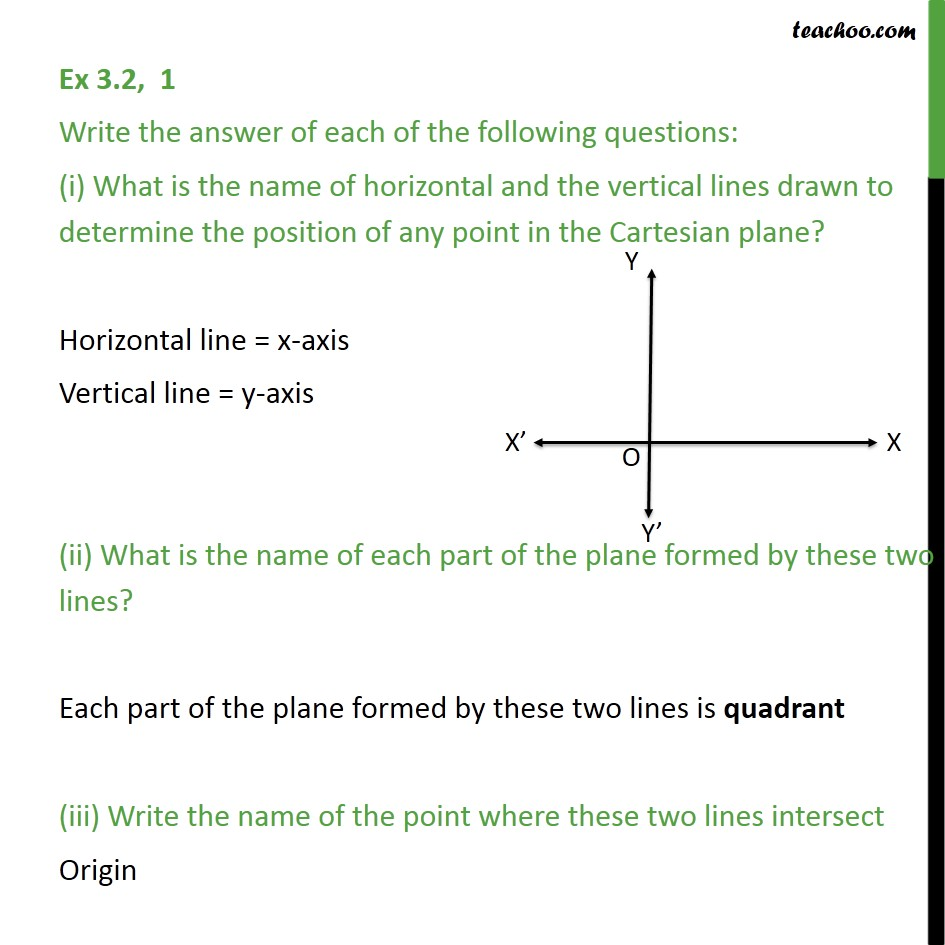Ex 3.2

Chapter 3 Class 9 Coordinate Geometry
Serial order wiseLearn in your speed, with individual attention - Teachoo Maths 1-on-1 Class

### Transcript

Ex 3.2, 1 Write the answer of each of the following questions: (i) What is the name of horizontal and the vertical lines drawn to determine the position of any point in the Cartesian plane? Horizontal line = x-axis Vertical line = y-axis (ii) What is the name of each part of the plane formed by these two lines? Each part of the plane formed by these two lines is quadrant (iii) Write the name of the point where these two lines intersect Origin